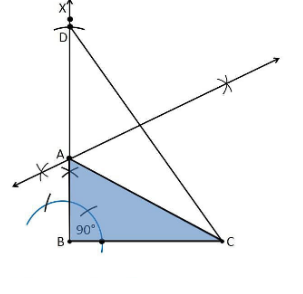Guru

# Construct a right triangle whose base is 12cm and sum of its hypotenuse and other side is 18 cm. Q.5

• 0

What is the simplest way for solving the question of class 9th of exercise 11.2  of math of question no.5  give me the best and simple way for solving this question in simple way Construct a right triangle whose base is 12cm and sum of its hypotenuse and other side is 18 cm.

Share

1. Construction Procedure:

The steps to draw the triangle of given measurement is as follows:

1. Draw a line segment of base BC = 12 cm

2. Measure and draw ∠B = 90° and draw the ray BX

3. Take a compass and measure AB+AC = 18 cm.

4. With B as centre and draw an arc at the point be D on the ray BX

5. Join DC

6. Now draw the perpendicular bisector of the line CD and the intersection point is taken as A.

7. Now join AC

8. Therefore, ABC is the required triangle.• 0